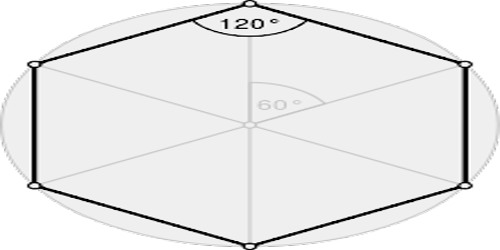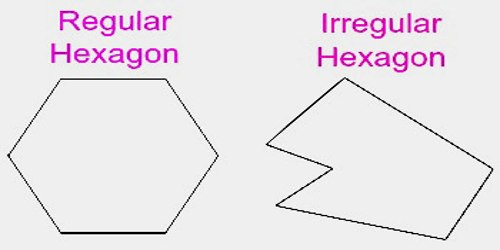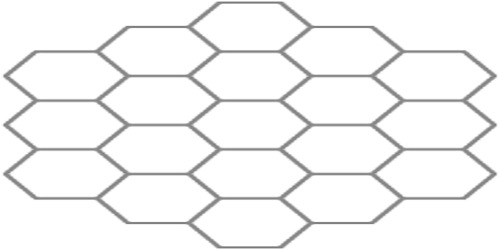Hexagon

Definition

Hexagon is a flat shape, all in one plane, with six sides all of equal length. Each of the six angles measures 120 degrees and the total of the internal angles of any hexagon is 720°. It can be cut into six equilateral triangles, all the same size. It has six rotational symmetries and six reflection symmetries, making up the dihedral group D6.The perimeter of a hexagon is the sum of the lengths of all the sides. All the sides are the same length, so that’s the same as the length of one side multiplied by six.

The properties of hexagons are numerous and interesting. At first glance, several facts about them stand out:

• Hexagons are one of only three regular polygons to tessellate the Euclidean plane, along with squares and triangles.
• The hexagonal tessellation is combinatorially identical to the close packing of circles on a plane.
• Hexagons are the only regular polygon that can be subdivided into another regular polygon.
• In a related fact, hexagons are the unique regular polygon such that the distance between the center and each vertex is equal to the length of each side.
• Hexagons are the first polygons, when ascending by number of sides that do not form the faces of a regular convex polyhedron in Euclidean space. The three polygons with fewer sides compose the surfaces of the five platonic solids, but no polygon with six or more sides can be employed for this purpose. A consequence of this is that no regular polytope, in any dimension, has hexagonal faces though many have hexagon-like or hexagonally-symmetric vertices or other elements.
• Hexagons are third-order permutohedra, meaning each vertex of a hexagon can be described with Cartesian coordinates using one of six permutations of the numbers 1, 2, and 3. Such a hexagon would lie on a plane consisting of all points with coordinates that add up to 6, and would bisect a cube of unit length 2 between coordinates 1 and 3.Types of Hexagon

Regular Hexagons – A hexagon is an example of a polygon, or a shape with many sides. Hex is a Greek prefix which means ‘six.’ A regular hexagon has six sides that are all congruent, or equal in measurement. A regular hexagon is convex, meaning that the points of the hexagon all point outward. All of the angles of a regular hexagon are congruent and measure 120 degrees. This means the angles of a regular hexagon add up to 720 degrees, or 6 times 120. A regular hexagon can also be created as a truncated equilateral triangle, with Schläfli symbol t{3}. Seen with two types (colors) of edges, this form only has D3 symmetry.

Irregular Hexagons – Irregular hexagons can look quite different. An irregular hexagon also has six sides, but they’re not of equal length. The points of irregular hexagons can point inward or outward. When the points of a hexagon point inward, even if only one point is inward, then the hexagon is considered a concave hexagon.Tesselations by Hexagons

In addition to the regular hexagon, which determines a unique tessellation of the plane, any irregular hexagon which satisfies the Conway criterion will tile the plane. A related property of hexagonal tessellation is that it creates no diagonal edges. In a square tessellation, every square connects to one square directly on each side and one square diagonally on each vertex, for eight total neighboring squares, and in a triangular tessellation every triangle connects to one triangle on each side, and three triangles diagonally on each vertex, for a total of twelve neighboring triangles. In addition, as hexagons can be divided into equilateral triangles, and equilateral triangles can be combined into hexagons, the triangular tessellation can be considered a simple subdivision of the hexagonal, or vice versa.

A final advantage of hexagonal tessellation or hexagonal lattices worth considering is its compressive and tensile strength and efficiency when compared to other tessellatory options.

Reference: